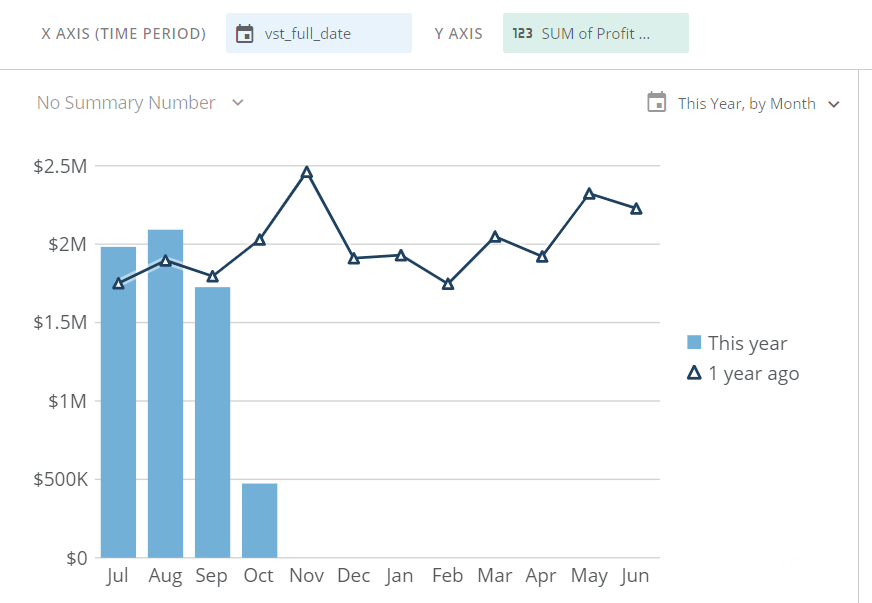# Compare two different metrics year over year

Hi - I'm wanting to create a card that compares two different metrics year over year.  Specifically, I want to compare projected profit contribution for the current fiscal year to date to actual profit contribution from the same period last year.

I currently have a simple p-o-p card to which I'd like to add a second bar with the projected profit contribution.

Is there any way to do this?

Thanks in advance for any help!

Laurie• I would approach this with a different chart type and beastmodes.

You can create beastmodes for 2017 Actual Profit and 2018 Actual Profit:

`2017 Actual Profit`

ifnull(SUM(CASE WHEN YEAR(`date field`) = 2017 THEN `actual profit field`
END),0)

`2018 Actual Profit`

ifnull(SUM(CASE WHEN YEAR(`date field`) = 2018 THEN `actual profit field`
END),0)

Then do the same for Projected profit:

`2017 Actual Profit`

ifnull(SUM(CASE WHEN YEAR(`date field`) = 2017 THEN `projected profit field`
END),0)

`2018 Actual Profit`

ifnull(SUM(CASE WHEN YEAR(`date field`) = 2018 THEN `projected profit field`
END),0)

for the graph, you will also want a month name field:

MONTHNAME(`date field`)

and month number (for sorting)

MONTH(`date field`)

You can then build a "Line + Grouped Bar" visualization.

X-axis would be the Month name beastmode

Y Axis is the first value that you want as a line (`2017 Actual Profit`)

Series:

The first field to add to the series is the second value you want as a line (`2017 Projected Profit`)

The next field is your first bar (`2018 Actual Profit`)

Then (`2018 Projected Profit`)

You will then want to sort by the month # field to get the month names in the right order

In chart Properties you will need to change the general setting "Series on Left Scale" to 2

I might suggest looking at running totals for the bars and lines as well unless the actual monthly performance is more important or informative.

Apologies, I didn't have the time to snap pictures for this post.  Let me know if you have more questions and I will try to help more

______________________________________________________________________________________________
“There is a superhero in all of us, we just need the courage to put on the cape.” -Superman
______________________________________________________________________________________________